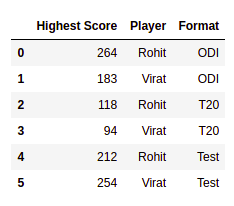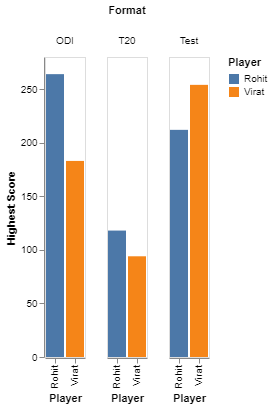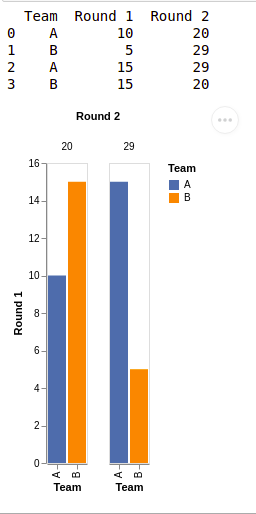# Create Grouped Bar Chart using Altair in Python

• Last Updated : 16 Mar, 2021

Grouped bar charts are a handy tool to represent our data when we want to compare multiple sets of data items one against another. To make a grouped bar chart, we require at least three rows of three columns of data in our dataset. The three columns can be used as- one for values, one for series, and one for categories. In a grouped bar chart, the data values present in a series are represented side by side and are grouped under specific categories so that their values can be represented accordingly over the axis.  All the data values belonging to a specific series will always be represented with the same color across all the categories.

Let’s take an example, suppose, we want to compare the runs made by two players across three formats. Here, runs scored by the players act as values, Player name acts as a series and the format of the game acts as the categories. Always, the runs scored by each player will have the same color representation across the different formats.

In this article, we will learn to create a grouped bar chart using Altair library in Python.

As we discussed, we need at least three rows/columns, we will begin with importing necessary libraries and then creating a dataset having three columns using pandas library.

Example 1:

## Python

 `import` `altair as alt ` `import` `pandas as pd ` ` `  `# creating a custom dataframe ` `data ``=` `pd.DataFrame([[``264``, ``'Rohit'``, ``'ODI'``],  ` `                     ``[``183``, ``'Virat'``, ``'ODI'``],  ` `                     ``[``118``, ``'Rohit'``, ``'T20'``],  ` `                     ``[``94``, ``'Virat'``, ``'T20'``], ` `                     ``[``212``, ``'Rohit'``,``'Test'``], ` `                     ``[``254``, ``'Virat'``,``'Test'``]], ` `                     ``columns``=``[``'Highest Score'``, ``'Player'``, ``'Format'``]) ` ` `  `print``(data)`

Output:Now, that we have a dataset containing three columns, where we want to compare the highest score (values) of two players (series) across different formats (categories).

## Python

 `gp_chart ``=` `alt.Chart(data).mark_bar().encode( ` `  ``alt.Column(``'Format'``), alt.X(``'Player'``), ` `  ``alt.Y(``'Highest Score'``, axis``=``alt.Axis(grid``=``False``)),  ` `  ``alt.Color(``'Player'``)) ` ` `  `gp_chart.display() `

Output:We can plot a group chart by calling the alt.Chart() method present inside Altair library. We want our categories to be displayed as the columns so, we can pass Format inside the alt.Column() method, our series (players) will be represented on the x-axis hence we pass Player in the alt.X() field, and our values (runs) should be displayed on y-axis so we pass Highest Score inside the alt.Y() field. Each player should have same color in all formats so, we pass Player inside the alt.Color() method.

Example 2:

## Python3

 `# importing package ` `import` `altair as alt ` `import` `pandas as pd ` ` `  `# create data ` `data ``=` `pd.DataFrame([[``'A'``, ``10``, ``20``], ` `                     ``[``'B'``, ``5``, ``29``], ` `                     ``[``'A'``, ``15``, ``29``], ` `                     ``[``'B'``, ``15``, ``20``]], ` `                    ``columns``=``[``'Team'``, ``'Round 1'``, ``'Round 2'``]) ` `# view data ` `print``(data) ` ` `  `gp_chart ``=` `alt.Chart(data).mark_bar().encode( ` `    ``alt.Column(``'Round 2'``), alt.X(``'Team'``), ` `    ``alt.Y(``'Round 1'``, axis``=``alt.Axis(grid``=``False``)), ` `    ``alt.Color(``'Team'``)) ` ` `  `gp_chart.display() `

Output:My Personal Notes arrow_drop_up
Recommended Articles
Page :